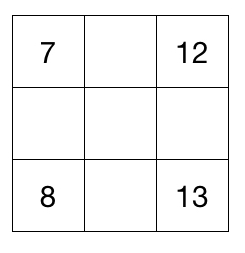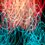# Magic Square Minimum Information

In this problem, I gave a partially completed magic square and had people figure out what the rest of the information is.That led me to think about the minimum amount of information that we need to provide, in order to determine the magic square.

I approach the problem in the following way:

• There are 10 unknowns - 9 values of the squares, 1 of the magic sum
• We have 8 equations - 3 horizontal, 3 vertical, 2 diagonal
• The system of equations isn't linear independent because the 3 horizontal sum up to the same as the 3 vertical. But I believe that dropping one of these equations is sufficient for linear independence, so we have 7 equations.
• If so, we then have a unique solution when there are 7 unknowns, which means that we need to provide information on 3 values.
• To show that this is optimal, in the above image, if we're given any 3 of those 4 values, we can uniquely determine the magic square.

And how about the $4 \times 4$ case?Note by Chung Kevin
3 years, 9 months ago

This discussion board is a place to discuss our Daily Challenges and the math and science related to those challenges. Explanations are more than just a solution — they should explain the steps and thinking strategies that you used to obtain the solution. Comments should further the discussion of math and science.

When posting on Brilliant:

• Use the emojis to react to an explanation, whether you're congratulating a job well done , or just really confused .
• Ask specific questions about the challenge or the steps in somebody's explanation. Well-posed questions can add a lot to the discussion, but posting "I don't understand!" doesn't help anyone.
• Try to contribute something new to the discussion, whether it is an extension, generalization or other idea related to the challenge.

MarkdownAppears as
*italics* or _italics_ italics
**bold** or __bold__ bold
- bulleted- list
• bulleted
• list
1. numbered2. list
1. numbered
2. list
Note: you must add a full line of space before and after lists for them to show up correctly
paragraph 1paragraph 2

paragraph 1

paragraph 2

[example link](https://brilliant.org)example link
> This is a quote
This is a quote
    # I indented these lines
# 4 spaces, and now they show
# up as a code block.

print "hello world"
# I indented these lines
# 4 spaces, and now they show
# up as a code block.

print "hello world"
MathAppears as
Remember to wrap math in $$ ... $$ or $ ... $ to ensure proper formatting.
2 \times 3 $2 \times 3$
2^{34} $2^{34}$
a_{i-1} $a_{i-1}$
\frac{2}{3} $\frac{2}{3}$
\sqrt{2} $\sqrt{2}$
\sum_{i=1}^3 $\sum_{i=1}^3$
\sin \theta $\sin \theta$
\boxed{123} $\boxed{123}$

Sort by:

Actually, there is only one answer.

Let $m_{1}$ and $m_{2}$ denote the sums of the middle row and middle column, respectively, of a 3 x 3 square array of numbers. Let $c _{1}$ and $c_{2}$ denote the sums of the main diagonal and secondary diagonal, respectively. Lastly, let S denote the sum of all nine elements of the square. Then if x denotes the centre element of the square,

$m_{1}+m_{2}+c_{1}+c_{2} = S + 3x$

Suppose the common sum is r,
x= r/3

Now $a + r/3 + b = r$ , where a and b are elements in the square containing the middle element x,

Therefore, $a + b = 2r/3 = 2x$

=> a,x,b are in Arithmetic progression

That means the elements of diagonals and middle row and column are in AP.

Let the square be like this

7 | y | 12

w | x | t

8 | z | 13

$19+y=21+z$ $=> y=2+z$

$2x = y+z$ $=>x= z+1$

$15+w = 25 +t$ $=> w=10+t$

$2x = w+t$ $=> x=5+t$ => $z=4+t$

$x=(12+8)/2$ = $(7+13)/2 =10$

Which gives us the square

7 | 11 | 12

15 | 10 | 5

8 | 9 | 13

with common sum equal to 30.There is no other answer, there may be more solutions but answer is one only.

- 2 years, 1 month ago

You had most of it. Just note that

1. Enclose the code in $\backslash( \quad \backslash)$ instead of  .
2. Place the entire equation in the brackets, not just certain parts.

I've edited the first few equations so that you can take a look at it.

Staff - 2 years ago

Thanks

- 2 years ago

Some pointers to note

1. To start a new line, leave 3 spaces at the end of the line.
2. To get the implication sign $(\Rightarrow)$, you can use \Rightarrow instead of =>. For more examples of code, see Math formatting guide.

Staff - 2 years ago

Sir, how can we make a box around the text,

just as done here to enclose the words " what is $\displaystyle \lim_{n \to \infty} A^n$"?

Start a new line with ">" at the start. In the formatting guide, it's the Markdown code of "> This is a quote". I've edited your comment so you can see how it's done.

Staff - 2 years ago

Can you explain your third dot point?

- 3 years, 6 months ago

If the magic square was

 A B C D E F G H I

Then we know that $S = A + B + C$, $S = D + E + F$, $S = G + H + I$ from the horizontal rows.

If we are also told that $S = A + D+ G$ and $S = B + E + H$, then we can conclude that $C + F + I = ( A + B + C ) + ( D + E + F ) + ( G + H + I ) - ( A + D+ G) - ( B + E + H ) = S + S + S - S - S = S$.
As such, this equation isn't "necessary", and we can drop it.

- 3 years, 6 months ago

I think I found the general solution for such square ، the formul for all the possible numbers which are satisfy the conditions of the square , all the possible numbers up to the infinity which give you same summation in all directions

 M M+4 M+5 M+8 M+3 M - 2 M+1 M+2 M+6

Notice that in all directions the summation will be 3M+9 which is the unique relation for the unique arrangement to have same summation in all direction Put it in Excel program to see every thing ، put M= - 3 and see how beautiful the symmetry of the numbers when you get zero summation in all directions ....

- 3 years, 7 months ago

Not all magic squares will be like that though all values of M will generate a magic square..

- 3 years, 7 months ago

Yes ....... but under this condition to have the same summation in all directions I think there is No other arrangement than this ....

Thank you ...

- 3 years, 7 months ago

Consider this;
3, 15, 12,
19,10 ,1,
8, 5, 17,

- 3 years, 7 months ago

you are right my friend ، this is approval that my theory is wrong ، hhhhhhhhhhhhhhh ، thank you ، I appreciate that ....

- 3 years, 7 months ago

With the added assumption that the numbers must be consecutive integers, I believe there is only 1 solution up to rotation / reflection.

Staff - 3 years, 7 months ago

Actually it depends on the value of M ، just insert M and see the corresponding set of values ...if M is integer number the set of corresponding numbers will be integer also ....

Thankfx.....

- 3 years, 7 months ago

I feel that for any nxn magic square, you only need n clues to get unique solution.

- 3 years, 8 months ago

The $4 \times 4$ magic square will need a lot more than 4 clues.

- 3 years, 8 months ago

@Zee Ell also mentioned that "Side sum is thrice of center square", which follows from summing up the 2 diagonals, the center row and the center column, and then subtracting the 3 rows.

This implies that we have 1 more equation in that system which is useless. Hence, it's possible for the magic square to just supply 3 numbers (like if we knew the entries in the left most column), which would then give us the center square and hence everything else.

Thanks!

- 3 years, 9 months ago

Sir, if negative values are allowed; then I "THINK" that if the values of the central square and any 2 adjacent corners is given we can solve for a unique magic square. I would like you to make a random magic square and omit all values except three; the central and any two adjacent corners; then I will try to solve and we may verify this.

- 3 years, 9 months ago

Negative values are allowed, as are fractional / decimal values. It shouldn't affect the square, because we can just "add k" to all terms.

I posted this problem that was inspired by Zee Ell's comment.

- 3 years, 9 months ago